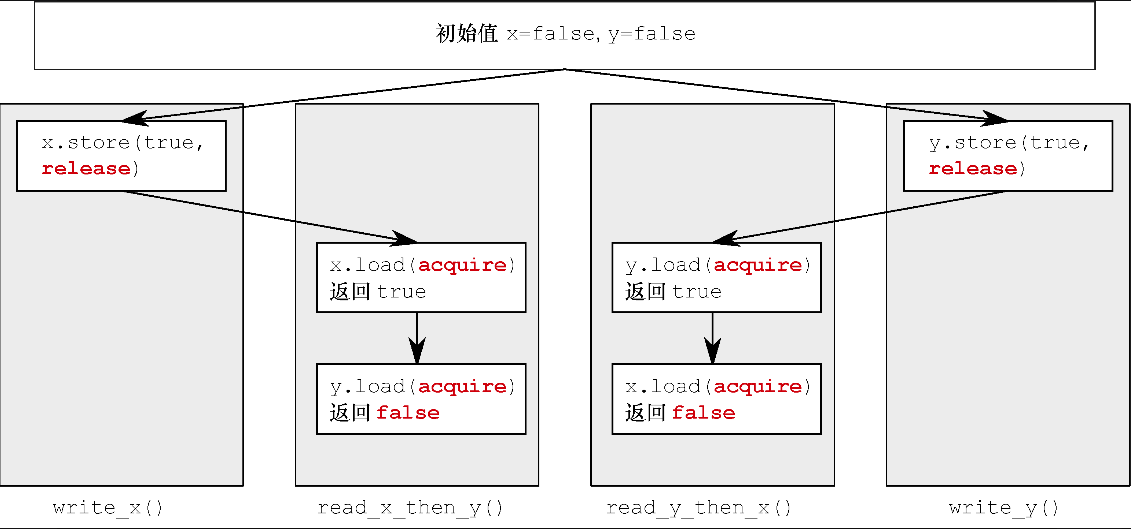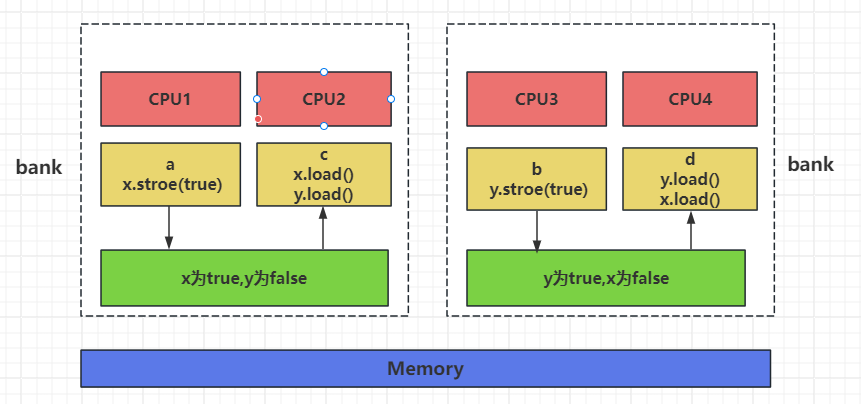# 恋恋风辰的官方博客

## 线程可见顺序

``#include <iostream>``#include <atomic>``#include <thread>``#include <cassert>``std::atomic<bool> x, y;``std::atomic<int> z;``void write_x()``{``    x.store(true, std::memory_order_release); //1``}``void write_y()``{``    y.store(true, std::memory_order_release); //2``}``void read_x_then_y()``{``    while (!x.load(std::memory_order_acquire));``    if (y.load(std::memory_order_acquire))   //3``        ++z;``}``void read_y_then_x()``{``    while (!y.load(std::memory_order_acquire));``    if (x.load(std::memory_order_acquire))   //4``        ++z;``}``

``void TestAR()``{``    x = false;``    y = false;``    z = 0;``    std::thread a(write_x);``    std::thread b(write_y);``    std::thread c(read_x_then_y);``    std::thread d(read_y_then_x);``    a.join();``    b.join();``    c.join();``    d.join();``    assert(z.load() != 0); //5``    std::cout << "z value is " << z.load() << std::endl;``}``

2 之后d线程读取时，如果保证执行到4处说明y为true，等d线程执行4处代码时x必然为true。
3 他们的理解是如果x先被store为true，y后被store为true，c线程看到y为false时x已经为true了，那么d线程y为true时x也早就为true了，所以z一定会执行加加操作。a执行`x.store(true)`先被线程c读取，而此时线程b对y的store还没有被c读取到新的值，所以此时c读取的x为true，y为false。

## 栅栏

``#include <atomic>``#include <thread>``#include <assert.h>``std::atomic<bool> x,y;``std::atomic<int> z;``void write_x_then_y()``{``    x.store(true,std::memory_order_relaxed); // 1``    y.store(true,std::memory_order_relaxed);   // 2``}``void read_y_then_x()``{``    while(!y.load(std::memory_order_relaxed));  // 3``    if(x.load(std::memory_order_relaxed))  // 4``        ++z;``}``int main()``{``    x=false;``    y=false;``    z=0;``    std::thread a(write_x_then_y);``    std::thread b(read_y_then_x);``    a.join();``    b.join();``    assert(z.load()!=0);  //5``}``

``void write_x_then_y3()``{``    x.store(true, std::memory_order_relaxed); // 1``    y.store(true, std::memory_order_release);   // 2``}``void read_y_then_x3()``{``    while (!y.load(std::memory_order_acquire));  // 3``    if (x.load(std::memory_order_relaxed))  // 4``        ++z;``}``

``void write_x_then_y_fence()``{``    x.store(true, std::memory_order_relaxed);  //1``    std::atomic_thread_fence(std::memory_order_release);  //2``    y.store(true, std::memory_order_relaxed);  //3``}``void read_y_then_x_fence()``{``    while (!y.load(std::memory_order_relaxed));  //4``    std::atomic_thread_fence(std::memory_order_acquire); //5``    if (x.load(std::memory_order_relaxed))  //6``        ++z;``}``

``void TestFence()``{``    x = false;``    y = false;``    z = 0;``    std::thread a(write_x_then_y_fence);``    std::thread b(read_y_then_x_fence);``    a.join();``    b.join();``    assert(z.load() != 0);   //7``}``

7处的断言也不会触发。我们可以分析一下，

2处能保证1处的指令先于2写入内存，进而”1 happens-before 6”, 1的结果会同步给 6

## 总结

https://space.bilibili.com/271469206/channel/collectiondetail?sid=1623290

https://gitee.com/secondtonone1/boostasio-learn/tree/master/concurrent/day13-fence

#### 热门文章

1. 解密定时器的实现细节

喜欢(566) 浏览(1340)
2. C++ 类的继承封装和多态

喜欢(588) 浏览(1859)
3. Linux环境搭建和编码

喜欢(594) 浏览(3732)
4. windows环境搭建和vscode配置

喜欢(587) 浏览(1496)
5. slice介绍和使用

喜欢(521) 浏览(1448)

#### 最新评论

1. 构造函数 secondtonone1:构造函数是类的基础知识，要着重掌握
2. visual studio配置boost库 Mr.test:visual studio时 应该使用  \b2.exe toolset=msvc”  🤡
3. 类和对象 陈宇航:支持！！！！
4. slice介绍和使用 恋恋风辰:切片作为引用类型极大的提高了数据传递的效率和性能，但也要注意切片的浅拷贝隐患，算是一把双刃剑，这世间的常态就是在两极之间寻求一种稳定。
5. Linux环境搭建和编码 恋恋风辰:Linux环境下go的安装比较简单，可以不用设置GOPATH环境变量，后期我们学习go mod 之后就拜托了go文件目录的限制了。

#### 个人公众号#### 个人微信